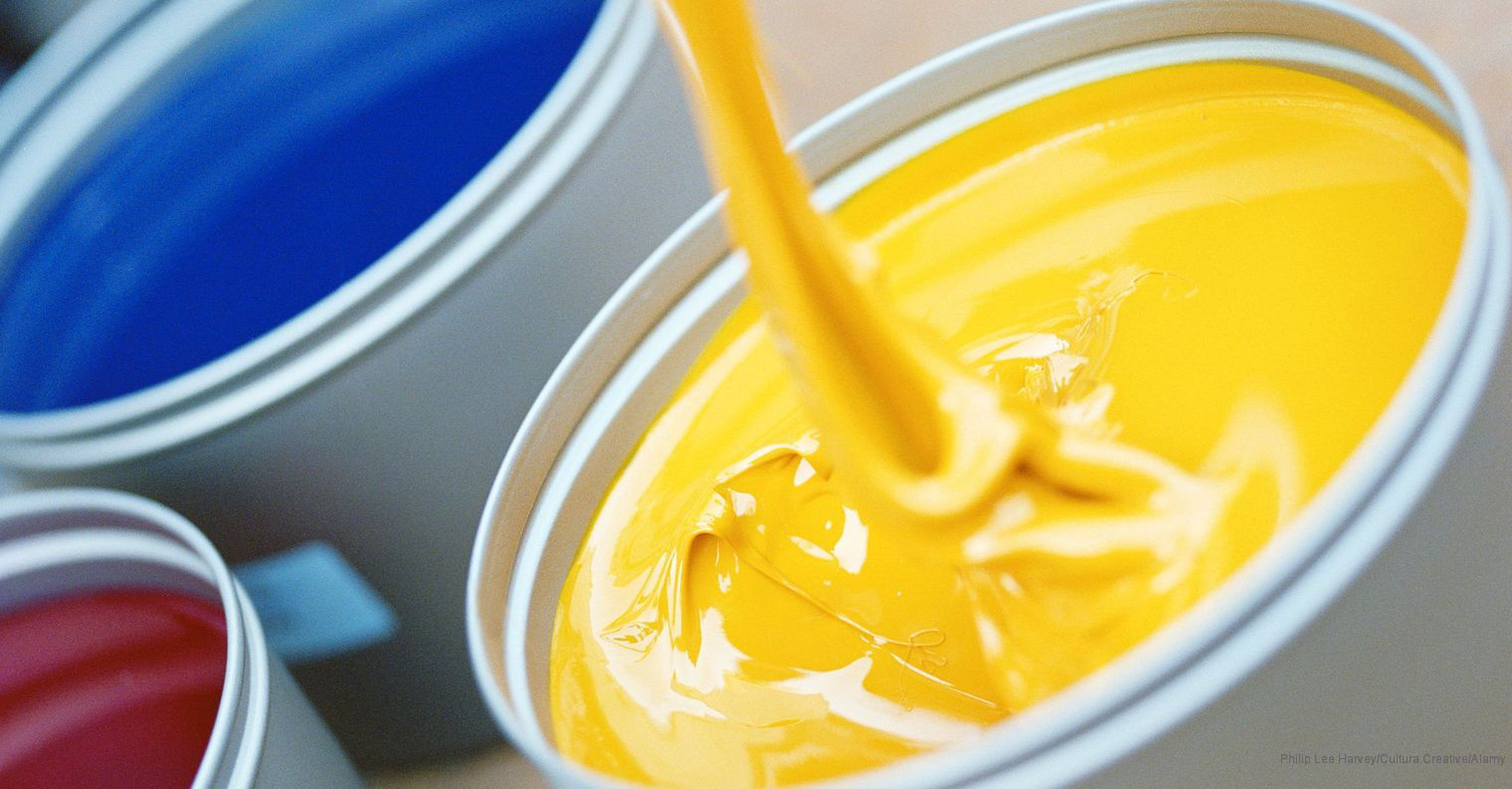# Expressing Ratios Numerically# Paint

Mia used the following reasoning to determine which paint is redder:

"I think it will be difficult to compare the two ratios in the problem because the numbers are so large. Maybe if I find equivalent ratios that have smaller numbers, it will be easier to solve the problem.

"The ratio of red to yellow in the Sunset paint is $\frac{24}{16}$. This ratio is equivalent to the ratio $\frac{3}{2}$.

"The ratio of red to yellow in the Apple paint is $\frac{48}{40}$. This ratio is equivalent to the ratio $\frac{6}{5}$.

"It’s easy to compare $\frac{3}{2}$ and $\frac{6}{5}$. I know that $\frac{3}{2}$ is greater than $\frac{6}{5}$. So, the Sunset paint is redder than the Apple paint."

• Talk to your partner about Mia's reasoning. Why is it more difficult to compare $\frac{24}{16}$ and $\frac{48}{40}$ than it is to compare $\frac{3}{2}$ and $\frac{6}{5}$?## Opening

Compare ratios using the simplest form of each ratio.

# Find Equivalent Paint Ratios

Work with a partner.

• Take turns finding and grouping cards that represent the same ratio of red paint to yellow paint.
• Explain to your partner how you know that the cards represent the same ratio.
• Your partner should either agree with your explanation or challenge it if your explanation is not correct, clear, or complete.
• When you have finished grouping all the cards in a way that you both agree on, arrange the sets of cards in order from least to greatest (the least red to the most red).

INTERACTIVE: Card Sort

# Prepare a Presentation

You arranged your sets of paint ratio cards from least to greatest in Task 3.

• Show how you grouped the cards and how you put the sets in order.
• Include an explanation of the method(s) you used.

# Challenge Problem

A painter mixes 15-fluid-ounce samples of red, yellow, and brown paint in the ratio of 1 to 3 to 2 in order to check that the resulting color is right for his mural. This sample mixture contains 90 fluid ounces (6 × 15 fluid ounces).

• For the mural, the painter needs 360 fluid ounces of paint in the same ratio (1:3:2). How many more fluid ounces of each color does he need?

How could you put the ratios on the cards in the same format to make them easier to compare?

# Ways of Thinking: Make Connections

Take notes as your classmates present their methods for putting the ratio cards into sets of equal ratios and ordering the sets.

• Did you and your partner have any disagreements about which cards represent the same ratio? If so, how did you resolve your disagreement?
• Do you think simplifying ratios that are represented as fractions is a good strategy? Why or why not?
• What do you think is the easiest way to tell whether two ratios are equivalent?
• Did you rewrite any of the ratios to help you determine how to arrange them from least to greatest? If so, how did you rewrite the ratios?

# Summary of the Math: Ratios in Simplest Form

Write a summary about using the simplest form of ratios and comparing ratios.

• Do you discuss why it is often easier to work with the simplest form of a ratio?
• Do you explain how to find the simplest form of a ratio?
• Do you describe how to compare and order ratios?

One way that I could figure out the relationship between the ratio 3:2 and the fraction $\frac{27}{18}$ is to …